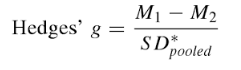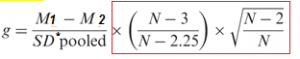# Hedges’ g: Definition, Formula

Effect Size > Hedges’ g

## What is Hedges’ g?

Hedges’ g is a measure of effect size. Effect size tells you how much one group differs from another—usually a difference between an experimental group and control group.

Hedges’ g and Cohen’s d are extremely similar. Both have an upwards bias (an inflation) in results of up to about 4%. The two statistics are very similar except when sample sizes are below 20, when Hedges’ g outperforms Cohen’s d. Hedges’ g is therefore sometimes called the corrected effect size.

• For very small sample sizes (<20) choose Hedges’ g over Cohen’s d.
• For sample sizes >20, the results for both statistics are roughly equivalent.
• If standard deviations are significantly different between groups, choose Glass’s delta instead. Glass’s delta uses only the control group’s standard deviation (SDC).

## Formula

The Hedge’s g formula is:Where:

Need help with the formula? Check out our tutoring page!

The main difference between Hedge’s g and Cohen’s D is that Hedge’s g uses pooled weighted standard deviations (instead of pooled standard deviations).

A note on small sample sizes:
Hedges’ g (like Cohen’s d) is biased upwards for small samples (under 50). To correct for this, use the following formula:## Interpreting Results

A g of 1 indicates the two groups differ by 1 standard deviation, a g of 2 indicates they differ by 2 standard deviations, and so on. Standard deviations are equivalent to z-scores (1 standard deviation = 1 z-score).

## Rule of Thumb Interpretation

Cohen’s d and Hedges’ g are interpreted in a similar way. Cohen suggested using the following rule of thumb for interpreting results:

• Small effect (cannot be discerned by the naked eye) = 0.2
• Medium Effect = 0.5
• Large Effect (can be seen by the naked eye) = 0.8

Cohen did suggest caution when using this rule of thumb. The terms “small” and “large” effects can mean different things in different areas. For example, a “small” reduction in suicide rates is invaluable, where a “small” weight loss may be meaningless. Durlak (2009) suggests referring to prior studies to see of where your results fit into the bigger picture.

References:
Cohen, J. (1977). Statistical power analysis for the behavioral sciences. Routledge.
Durlak, J. (2009) How to Select, Calculate, and Interpret Effect Sizes. Journal of Pediatric Psychology. March: 34(9):917-28.
Ellis, P. (2010). The Essential Guide to Effect Sizes: Statistical Power, Meta-Analysis, and the Interpretation of Research Results.
Hedges, L. (1981). Distribution Theory for Glass’s Estimator of Effect Size and Related Estimators. Journal of Educational Statistics. Vol. 6, No. 2 (Summer, 1981), pp. 107-128. Entire PDF available for free from JSTOR.
Hedges L. V., Olkin I. (1985). Statistical methods for meta-analysis. San Diego, CA: Academic Press

CITE THIS AS:
Stephanie Glen. "Hedges’ g: Definition, Formula" From StatisticsHowTo.com: Elementary Statistics for the rest of us! https://www.statisticshowto.com/hedges-g/